# Setting up a Calculated Simple question in Moodle 2.3

Creating calculated questions in Moodle 2.3 can be quicker using the Calculated Simple question type in the Moodle Question Bank. If you have not reviewed the Calculated and Calculated multichoice tutorials, please do so as they will help with this tutorial. The following article will explain the steps for setting up the Calculated Simple question within Moodle.

### Creating a Simple Calculated question

2. Navigate to Front page settings > Question bank > Questions. Click Create a new question and select Calculated Simple and click Next.
3.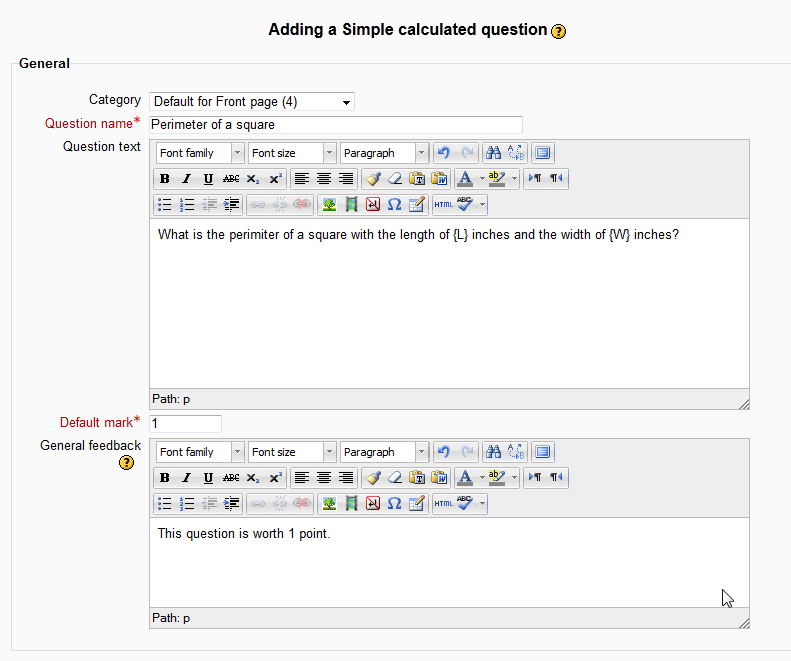On the Adding a Simple calculated question page, Fill in the General section. This is the same as step 4 of the Inserting a Calculated question in the Moodle Question Bank. In this tutorial, the question will be on the Perimeter of a square. The question will be as follows:

What is the perimeter of a square with the length of {L} inches and the width of {W} inches?

4.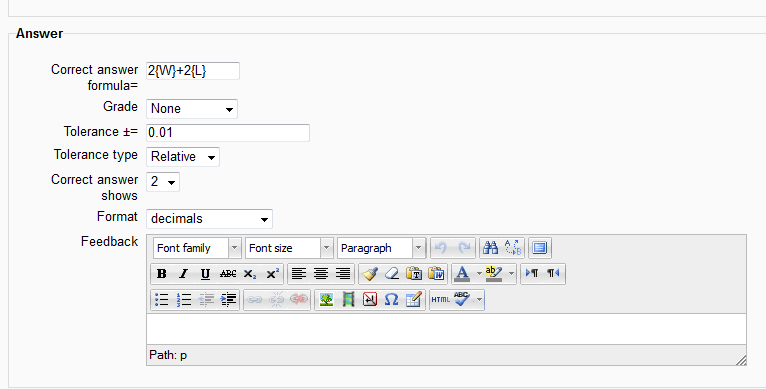In the Answer section, enter the formula in the Correct Answer Formula= box. For information on the tolerance see Working with the calculated question Tolerance in Moodle 2.3

Hint! Formulas should be created the same way they are entered into a calculator.

The answer formula for this question will be 2*{W}+2*{L}

Note! The grade of one of the answers should have a score of 100% so it is possible to get full marks for this question.

5.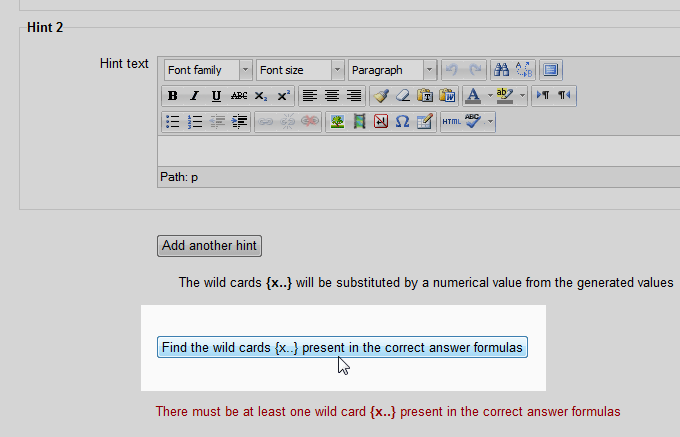Scroll down to after the Hint section to the Find the wild cards {x…} present in the correct answer formulas button. This feature is a part of the Simple Calculated question. Moodle will generate the Parameters for the questions based off of what was inputted in the Question text of the General section. Click the button.

6.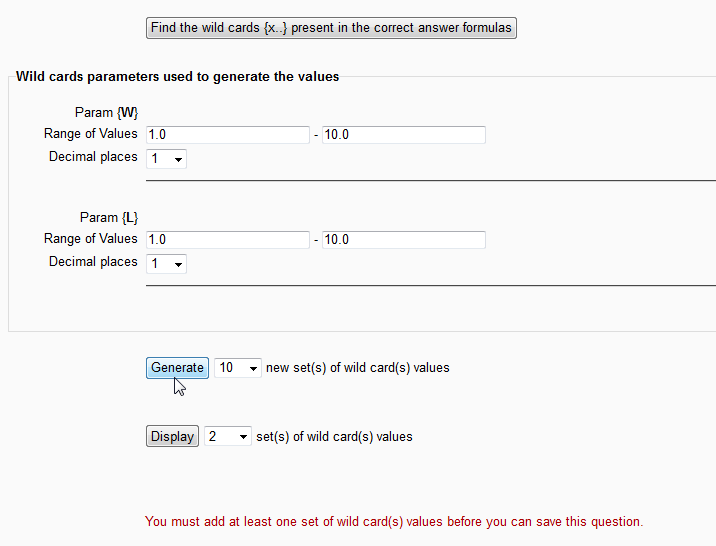Now the Wild cards parameters used to generate the valuesbox will appear. Here the Range of values and the Decimal places for both variables can be edited. Adjust the Range of Values for each wild card variable.

Set the quantity of wild cards to generate and how many to display. The Generate button will determine how many datasets to make. The Display button will display only the amount selected in the box. In this tutorial, Generate 10 and Display 2. Click Generate.

7.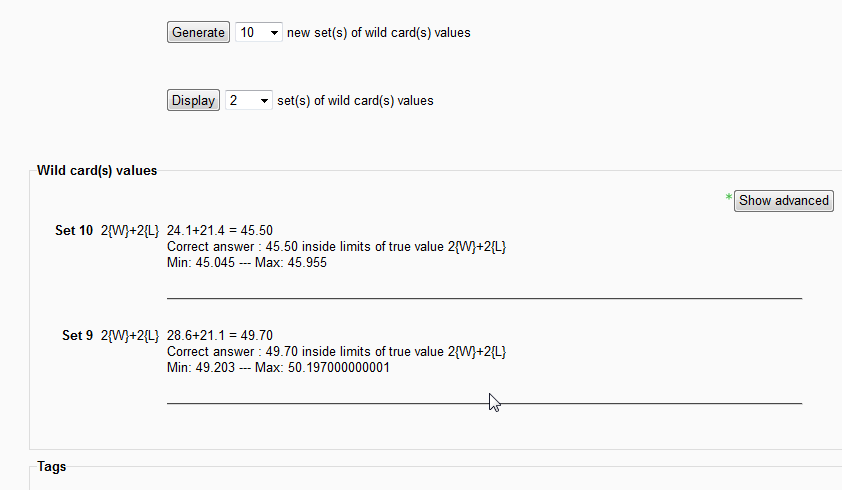The Wild card(s) values element will appear. Only 2 will display because the display was set to 2. Click Save changes.

8.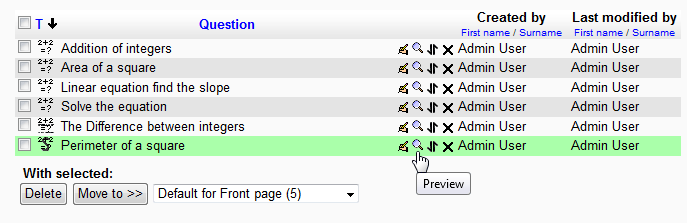Finally, Preview the question in the Question Bank.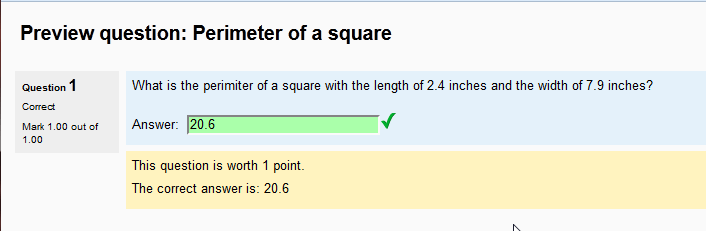When answering the question, because 10 Datasets were created. This question will be randomized with 10 different numbers and answers.

This concludes the article for Setting up a Calculated Simple question in Moodle 2.3. After understanding the Calculated questions, lets move to a simpler question type, the Essay question. Please check the next article on Creating an Essay question in the Moodle Question bank.InMotion Hosting Contributor Content Writer

InMotion Hosting contributors are highly knowledgeable individuals who create relevant content on new trends and troubleshooting techniques to help you achieve your online goals!

More Articles by InMotion Hosting